# RS Aggarwal Solutions Chapter 16 Coordinate Geometry Exercise 16C Class 10 Maths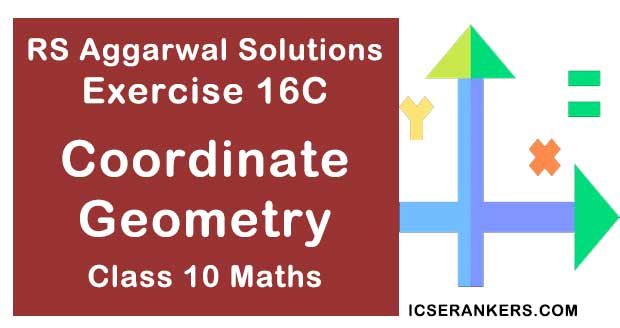Chapter Name RS Aggarwal Chapter 16 Coordinate Geometry Book Name RS Aggarwal Mathematics for Class 10 Other Exercises Exercise 16AExercise 16BExercise 16DMCQ Related Study NCERT Solutions for Class 10 Maths

### Exercise 16C Solutions

1. Find the area of ABC whose vertices are:

(i) A(1, 2), B(-2, 3) and C(-3, -4)

(ii) A(-5, 7), B(- 4, -5) and C(4, 5)

(iii) A(3, 8), B(-4, 2) and C(5, -1)

(iv) A(10, -6), B(2, 5) and C(-1, -3)

Solution

(i) A(1, 2) , B(-2, 3) and C(-3, -4) are the vertices of △ABC. Then,

(x1 = y, y1 = 2), (x2 = -2, y2 = -3) and (x3 = -3, y3 = -4)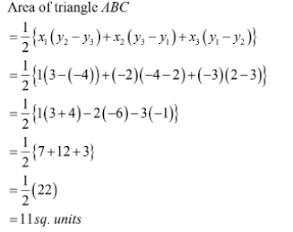(ii) A(-5, 7), B(-4, -5) and C(4, 5) are the vertices of △ABC. Then,

(x1 = -5, y1 = 7), (x2 = -4, y2 = - 5) and (x3 = 4, y3 = 5)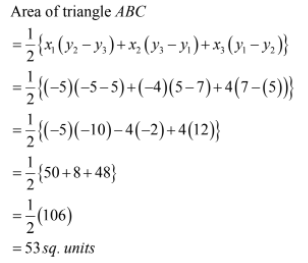(iii) A(3, 8), B(-4, 2) and C(5, -1) are vertices of △ABC. Then,

(x1 = 3, y1 = 8), (x2 = -4, y2 = 2) and (x3 = 5, y3 = -1)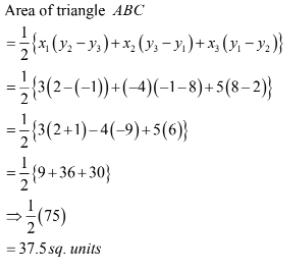(iv) A(10, -6), B(2, 5) and C(-1, -3) are the vertex of △ABC. Then,

(x1 = 10, y1 = -6), (x2 = 2, y2 = 5) and (x3 = -1, y3 = 3)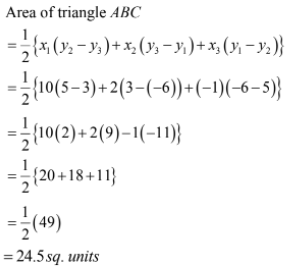2. Find the area of a quadrilateral ABCD whose vertices area A(3, -1), B(9, -5) C(14, 0) and D(9, 19).

Solution

By joining A and C, we get two triangles ABC and ACD.

Let

A(x1, y1) = A(3, -1), B(x2, y2) = B(9, -5), C(x3, y3) = C(14, 0) and D(x4, y4) = D(9, 19)

Then,

Area of △ABC = 1/2[x1(y2 – y3) + x2(y3 – y1) + x3(y1 – y2)]

= 1/2[3(-5 – 0) + 9(0 + 1) + 14(-1 + 5)]

= 1/2[-15 + 9 + 56]

= 25 sq. units.

Area of △ACD = 1/2[x1(y3 – y4) + x3(y4 – y1) + x4(y1 – y3)]

= 1/2[3(0 – 19) + 14(19 + 1) + 9(- 1 – 0)]

= ½[-57 + 280 – 9]

= 107 sq. units

So, the area of the quadrilateral is 25 + 107

= 132 sq. units.

3. Find the area of quadrilateral PQRS whose vertices are P(-5, -3), Q(-4, -6), R(2, -3) and S(1, 2).

Solution

By joining P and R, we get two triangles PQR and PRS.

Let P(x1, y1) = P(-5, -3), Q(x2, y2) = Q(-4, -6), R(x3, y3) = R(2, -3). Then,

S(x4, y4) = S(1, 2)

Area of △PQR = 1/2[x1(y2 – y3) + x2(y3 – y1) + x3(y1 – y2)]

= ½[-5(-6 + 3) – 4(-3 + 3) + 2(-3 + 6)]

= ½[15 – 0 + 6]

= 21/2 sq. units

Area of △PRS = 1/2[x1[(y3 – y4) + x3(y4 – y1) + x4(y1 – y3)]

= ½[-5 (-3 – 2) + 2(2 + 3) + 1(- 3 + 3)]

= ½[25 + 10 + 0]

= 35/2 sq. units.

So, the area of the quadrilateral PQRS is 21/2 + 35/2

= 28 sq. units units.

4. Find the area of quadrilateral ABCD whose vertices are A(-3, -1), B(-2, -4) C(4, -1) and D(3, 4)

Solution

By joining A and C, we get two triangles ABC and ACD.

Let A(x1, y1) = A(-3, -1), B(x2, y2) = B(-2, -4), C(x3, y3) = C(4, -1) and Then D(x4, y4) = D(3, 4)

Area of △ABC = ½[x1(y2 – y3) + x2(y3 – y1) + x3(y1 – y2)]

= ½[-3(-4 + 1) – 2(-1 + 1) + 4(-1 + 4)]

= ½[9 = 0 + 12]

= 21/2 sq. units.

Area of △ACD = ½(x1(y3 – y4) + x3(y4 – y1) + x4(y1 – y3)]

= ½[-3(-1 – 4) + 4(4 + 1) + 3(-1 + 1)]

= ½[15 + 20 + 0]

= 35/2 sq. units

So, the area of the quadrilateral ABCD is 21/2 + 35/2

= 28 sq. units

5. Find the area of quadrilateral ABCD whose vertices are A(-5, 7), B(-4, -5) C(-1, -6) and D(4, 5)

Solution

By joining A and C, we get two triangles ABC and ACD.

Let A(x1, y1) = A(-5, 7), B(x2, y2) = B(-4, -5), C(x3, y3) = C(-1, -6) and D(x4, y4) = D(4, 5)

Then,

Area of △ABC = ½[x1(y2 – y3) + x2(y3 – y1) + x3(y1 – y2)]

= ½[-5(-5 + 6) – 4(-6 – 7) – 1(7 + 5)]

= 1/2[- 5 + 52 – 12]

= 35/2 sq. units.

Area of △ACD = ½[x1(y3 – y4) + x3(y4 – y1) + x4(y1 – y3)]

= ½[-5(-6 – 5) – 1(5 – 7) + 4(7 + 6)]

= ½[55 + 2 + 52]

= 109/2 sq. units

So, the area of the quadrilateral ABCD is 35/2 + 109/2

= 72 sq. units.

6. Find the area of the triangle formed by joining the midpoints of the sides of the triangle whose vertices are A(2, 1) B(4, 3) and C(2, 5)

Solution

The verticals of the triangle are A(2, 1), B(4, 3) and C(2, 5)

Coordinates of midpoint of AB = P((x1, y1) = (2 + 4)/2, (1 + 3)/2 = (3, 2)

Coordinates of midpoint of BC = Q(x2, y2) = (4 + 2)/2, (3 + 5)/2 = (3, 4)

Coordinates of midpoint of AC = R(x3, y3) = (2 + 2)/2, (1 + 5)/2 = (2, 3)

Now,

Area of △PQR = ½[x2(y2 – y3) + x2(y3 – y1) + x3(y1 – y2)]

= ½[3(4 – 3) + 3(3 – 2) + 2(2 – 4)]

= ½[3 + 3 – 4]

= 1 sq. unit

Hence, the area of the quadrilateral triangle is 1 sq. unit.

7. A(7, -3), B(5, 3) and C(3, -1) are the vertices of a ABC and AD is its median. Prove that the median AD divides ABC into two triangles of equal areas.

Solution

The vertices of the triangle are A(7, -3), B(5, 3), C(3, -1)

Coordinates of D = (5 + 3)/2, (3 – 1)/2 = (4, 1)

For the area of the triangle ADC, let

A(x1, y1) = A(7, -3), D(x2, y2) = D(4, 1) and C(x3, y3) = C(3, -1). Then

Area of △ADC = 1/2[x1(y2 – y3) + x2(y3 – y1) + x3(y1 – y2)]

= ½[7(1 + 1) + 4(-1 + 3) + 3(-3 – 1)]

= ½[14 + 8 – 12]

= 5 sq. unit

Now, for the area of triangle ABD, let

A(x1, y1) = A(7, -3), B(x2, y2) = B(5, 3) and D(x3, y3) = D(4, 1). Then

Area of △ADC = ½[x1(y2 – y3) + x2(y3 – y1) + x3(y1 – y2)]

= ½[7(3 – 1) + 5(1 + 3) + 4(- 3 – 3)]

= ½[14 + 20 – 24]

= 5 sq. unit

Thus, Area (△ADC) = Area(△ABD) = 5 sq. units.

Hence, AD divides △ABC into two triangles of equal areas.

8. Find the area ABC with A(1, -4) and midpoints of sides through A being (2, -1) and (0, -1).

Solution

Let (x2, y2) and (x3, y3) be the coordinates of B and C respectively. Since, the coordinates of A are (1, -4), therefore

(1 + x2)/2 ⇒ x2 = 3

(-4 + y2)/2 = - 1

⇒ y2 = 2

(1 + x2)/2 = 0

⇒ x3 = -1

(-4 + y3)/2 = - 1

⇒ y3 = 2

Let A(x1, y1) = A(1, -4), B(x2, y2) = B(3, 2) and C(x3, y3) = C(-1, 2) Now

Area(△ABC) = ½[x1(y2 – y3) + x2(y3 – y1) + x3(y1 – y2)]

= ½[1(2 – 2) + 3(2 + 4) – 1(-4 – 2)]

= ½[0 + 18 + 6]

= 12 sq. units

Hence, the area of the triangle △ABC is 12 sq. units.

9. A(6, 1), B(8, 2) and C(9, 4) are the vertices of a parallelogram ABCD. If E is the midpoint of DC, find the area of ADE

Solution

Let(x, y) be the coordinates of D and (x’, y’) be the coordinates of E. Since, the diagonals of a parallelogram bisect each other at the same point, therefore

(x + 8)/2 = (6 + 9)/2 ⇒ x = 7

(y + 2)/2 = (1 + 4)/2 ⇒ y = 3

Thus, the coordinates of D are (7, 3) E is midpoint of DC, therefore

x’ = (7 + 9)/2

⇒ x’ = 8

y’ = (3 + 4)/2

⇒ y’ = 7/2

Thus, the coordinates of E are (8, 7/2)

Let A(x1, y1) = A(6, 1), E(x2, y2) = E(8, 7/2) and D(x3, y3) = D(7, 3) Now

Area (△ABC) = ½[x1(y2 – y3) + x2(y3 – y1) + x3(y1 – y2)]

= ½[6(7/2 – 3) + 8(3 – 1) + 7(1 – 7/2)]

= ½[3/2]

= ¾ sq. unit

Hence, the area of the triangle △ADE is 3/4 sq. units.

10. If the vertices of ABC be A(1, -3), B(4, p) and C(-9, 7) and its area is 15 square units, find the values of p.

Solution

Let A(x1, y1) = A(1, -3), B(x2, y2) = B(4, p) and C(x3, y3) = C(-9, 7)

Now

Area of (△ABC) = ½[x1(y2 – y3) + x2(y3 – y1) + x3(y1 – y2)]

⇒ 15 = 1/2 [1(p – 7) + 4(7 + 3) – 9(-3 – p)]

⇒ 15 = ½[10p + 60]

⇒ |10p + 60| = 30

Therefore,

⇒ 10p + 60 = -30 or 30

⇒ 10p = - 90 or – 30

⇒ p = -9 or -3

Hence, p = -9 or p = -3

11. Find the value of k so that the area of the triangle with vertices A(k + 1, 1), B(4, -3) and C(7, -k) is 6 square units.

Solution

Let A(x1, y1) = A(k + 1, 1), B(x2, y2) = B(4, -3) and C(x3, y3) = C(7, -k) Now

Area of (△ABC) = ½[x1(y2 – y1) + x2(y3 – y1) + x3(y1 – y2)]

⇒ 6 = ½[(k + 1)(-3 + k) + 4(-k – 1) + 7(1 + 3)]

⇒ 6 = ½[k2 – 2k – 3 – 4k – 4 + 28]

⇒ k2 – 6k + 9 = 0

⇒ (k – 3)2 = 0

⇒ k = 3

Hence, k = 3.

12. For what value of k(k > 0) is the area of the triangle with vertices (-2, 5), (k, -4) and (2k + 1, 10) equal to 53 square units ?

Solution

Let A(x1 = -2, y1 = 5), B(x= k, y2 = -4) and C(x3 = 2k + 1, y3 = 10) be the vertices of the triangle, So

Area (△ABC) = 1/2[x1(y– y3) + x2(y– y1) + x3(y1 – y2)]

⇒ 53 = ½[(-2)(-4 – 10) + k(10 – 5) + (2k + 1)(5 + 4)]

⇒ 53 = ½[28 + 5k + 9(2k + 1)]

⇒ 28 + 5k + 18k + 9 = 106

⇒ 37 + 23k = 106

⇒ 23k = 106 – 37 = 69

⇒ k = 69/23 = 3

Hence, k = 3

13. Show that the following points are collinear:

(i) A(2, -2), B(-3, 8) and C(-1, 4)

(ii) A(-5, 1), B(5, 5) and C(10, 7)

(iii) A(5, 1), B(1, -1) and C(11, 4)

(iv) A(8, 1), B(3, -4) and C(2, -5)

Solution

(i) Let A(x1 = 2, y1 = -2), B(x2 = -3, y2 = 8) and C(x= -1, y3 = 4) be the given points.

Now, x1(y– y1) + x2(y– y1) + x3(y1 – y2)

= 2(8 – 4) + (-3)(4 + 2) + (-1)(-2 – 8)

= 8 – 18 + 10

= 0

Hence, the given points are collinear.

(ii) Let A(x1 = -5, y1 = 1), B(x= 5, y= 5) and C(x3 = 10, y= 7) be the given points.

Now, x1(y– y3) + x2(y3 – y1) + x3(y– y2)

= (-5)(5 – 7) + 5(7 – 1) + 10(1 – 5)

= -5(-2) + 5(6) + 10(-4)

= 10 + 30 – 40

= 0

Hence, the given points are collinear.

(iii) Let A(x1 = 5, y1 = 1), B(x2 = 1, y2 = -1) and C(x3 = 11, y3 = 4) be the given points.

Now x1(y2 – y3) + x2(y3 – y1) + x3(y1 – y2)

= 5(-1 – 4) + 1(4 – 1) + 11(1 + 1)

= -25 + 3 + 22

= 0

Hence, the given points are collinear.

(iv) Let A(x1 = 8, y1 = 1), B(x2 = 3, y2 = -4) and C(x3 = 2, y3 = -5) be the given points.

Now, x1(y2 – y3) + x2(y3 – y1) + x3(y1 – y2)

= 8(-4 + 5) + 3(-5 – 1) + 2(1 + 4)

= 8 – 18 + 10

= 0

Hence, the given points are collinear.

14. Find the value of x for which points A(x, 2), B(-3, -4) and C(7, -5) are collinear.

Solution

Let A(x1, y1) = A(x, 2), B(x2, y2) = B(-3, -4) and C(x3, y3) = C(7, -5). So the condition for three collinear points is

x1(y2 – y3) + x2(y3 – y1) + x3(y1 – y2) = 0

⇒ x(-4 + 5) – 3(-5 – 2) + 7(2 + 4) = 0

⇒ x + 21 + 42 = 0

⇒ x = - 63

Hence, x = -63.

15. For what value of x are the points A(-3, 12), B(7, 6) and C(x, 9) are collinear.

Solution

A(-3, 12), B(7, 6) and C(x, 9) are the given points. Then:

(x1 = -3, y1 = 12), (x2 = 7, y2 = 6) and (x3 = x, y3 = 9)

It is given that points A, B and C are collinear. Therefore,

x1(y2 – y3) + x2(y3 – y1) + x3(y1 – y2) = 0

⇒ (-3)(6 – 9) + 7(9 – 12) + x(12 – 6) = 0

⇒ (-3)(-3) + 7(-3) + x(6) = 0

⇒ 9 – 21 + 6x = 0

⇒ 6x – 12 = 0

⇒ 6x = 12

⇒ x = 12/6 = 2

Therefore, when x = 2, the given points are collinear

16. For what value of y, are the points P(1, 4), Q(3, y) and R(-3, 16) are collinear ?

Solution

P(1, 4), Q(3, y) and R(-3, 16) are the given points. Then:

(x1 = 1, y1 = 4), (x2 = 3, y2 = y) and (x3 = -3, y3 = 16)

It is given that the points P, Q and R are collinear.

Therefore,

x1(y2 – y3) + x2(y3 – y1) + x3(y1 – y2) = 0

⇒ 1(y – 16) + 3(16 – 4) + (-3)(4 – y) = 0

⇒ 1(y – 16) + 3(16 – 4) + (-3)(4 – y) = 0

⇒ 1(y – 16) + 3(12) – 3(4 – y) = 0

⇒ y – 16 + 36 – 12 + 3y = 0

⇒ 8 + 4y = 0

⇒ 4y = - 8

⇒ y = -(8/4) = -2

When y = -2, the given points are collinear.

17. Find the value of y for which the points A(-3, 9),B(2, y) and C(4, -5) are collinear.

Solution

Let A(x1 = -3, y1 = 9), B(x2 = 2, y2 = y) and C(x3 = k, y3 = -5) be the given points

The given points are collinear if

x1(y2 – y3) + x2(y3 – y1) + x3(y1 – y2) = 0

⇒ (-3)(y + 5) + 2(-5 – 9) + 4(9 – y)= 0

⇒ -3y – 15 – 28 + 36 – 4y = 0

⇒ 7y = 36 – 43

⇒ y = -1

18. For what values of k are the points A(8, 1), B(3, -2k) and C(k, -5) collinear.

Solution

Let A(x1 = 8, y1 = 1), B(x2 = 3, y2 = -2k) and C(x3 = k, y3 = -5) be the given points

The given points are collinear if

x1(y2 – y3) + x2(y3 – y1) + x3(y1 – y2) = 0

⇒ 8(-2k + 5) + 3(- 5 – 1) + k(1 + 2k) = 0

⇒ - 16k + 40 – 18 + k + 2k2 = 0

⇒ 2k2 – 15k + 22 = 0

⇒ 2k2 – 11k – 4k + 22 = 0

⇒k(2k – 11) – 2(2k – 11) = 0

⇒ (k – 2)(2k – 11) = 0

⇒ k = 2 or k = 11/22

Hence, k = 2 or k = 11/22

19. Find a relation between x and y, if the points A(2, 1), B(x, y) and C(7, 5) are collinear.

Solution

Let A(x1 = 2, y1 = 1), B(x2 = x, y2 = y) and C(x3 = 7, y3 = 5) be the given points

The given points are collinear if

x1(y2 – y3) + x2(y3 – y1) + x3(y1 – y2) = 0

⇒ 2(y – 5) + x(5 – 1) + 7(1 – y) = 0

⇒ 2y – 10 + 4x + 7 – 7y = 0

⇒ 4x – 5y – 3 = 0

Hence, the required relation is 4x – 5y – 3 = 0

20. Find a relation between x and y, if the points A(x, y), B(-5, 7) and C(-4, 5) are collinear.

Solution

Let A(x1 = x, y1 = y), B(x2 = - 5, y2 = 7) and C(x3 = -4, y3 = 5) be the given points

The given points are collinear if

x1(y2 – y3) + x2(y3 – y1) + x3(y1 – y2) = 0

⇒ x(7 – 5) + (-5)(5 – y) + (-4)(y – 7) = 0

⇒ 7x – 5x – 25 + 5y – 4y + 28 = 0

⇒ 2y – 10 + 4x + 7 – 7y = 0

⇒ 2x + y + 3 = 0

Hence, the required relation is 2x + y + 3 = 0

21. Prove that the points A(a, 0), B(0, b) and C(1, 1) are collinear, if (1/a + 1/b) = 1.

Solution

Consider the points A(a, 0), B(0, b) and (x3 = 1, y3 = 1)

Here, (x1 = a, y1 = 0), (x2 = 0, y2 = b) and (x3 = 1, y3 = 1).

It is given that the points are collinear. So,

x1(y2 – y3) + x2(y3 – y1) + x3(y1 – y2) = 0

⇒a(b – 1) + 0(1 – 0) + 1(0 – b) = 0

⇒ ab – a – b = 0

Dividing the equation by ab:

⇒ 1 – 1/b – 1/a = 0

⇒ 1 – (1/a + 1/b) = 0

⇒ (1/a + 1/b) = 1

Therefore, the given points are collinear if (1/a + 1/b) = 1.

22. If the points P(-3, 9), Q(a, b) and R(4, -5) are collinear and a + b = 1, find the value of a and b.

Solution

Let A(x1 = 3, y1 = 9), B(x2 = a, y3 = b) and C(x3 = 4, y1 = -5) be the given points.

The given points are collinear if

x1(y2 – y3) + x3(y3 – y1) + x3(y1 – y2) = 0

⇒ (-3)(b + 5) + a(-5 – 9) + 4(9 – b) = 0

⇒ -3b – 15 – 14a + 36 – 4b = 0

⇒ 2a + b = 3

Now, solving a + b = 1 and 2a + b = 3, we get a = 2 and b = -1

Hence, a = 2 and b = -1

23. Find the area of ABC with vertices A(0, -1), B(2, 1) and C(0, 3). Also, find the area of the triangle formed by joining the midpoints of its sides. Show that the ratio of the areas of two triangles is 4: 1.

Solution

Let A(x1 = 0, y1 = -1), B(x2, y2 = 1) and C(x3 = 0, y3 = 3) be the given points. Then

Area (△ABC) = ½[x1(y2 – y3) + x2(y3 – y1) + x3(y1 – y2)]

= ½[0(1 – 3) + 2(3 + 1) + 0(- 1 – 1)]

= 1/2 × 8

= 4 sq. units

So, the area of the triangle △ABC is 4sq. units.

Let D(a1, b1), E(a2, b2) and F(a3, b3) be the midpoints of AB, BC and AC respectively

Then

a1 = (0 + 2)/2 = 1, b1 = (-1 + 1)/2 = 0

a2 = (2 + 0)/2 = 1, b2 = (1 + 3)/2 = 2

a3 = (0 + 0)/2 = 0, b3 = (-1 + 3)/2 = 1

Thus, the coordinates of D, E and F are D(a1 = 1, b1 = 0), E(a2 = 1, b2 = 2) and

F(a3 = 0, b3 = 1). Now

Area (△DEF) = ½[a1(b2 – b2) + a2(b3 – b1) + a3(b1 – b2)]

= ½[1(2 – 1) + 1(1 – 0) + 0(0 – 2)]

= ½[1 + 1 + 0]

= 1 sq. unit

So, the area of triangle △DEF is 1 sq. unit.

Hence, △ABC : △DEF = 4 :1